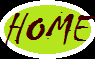## Wednesday, April 3, 2013

### Conditional Statements in PL/SQL || If || for || while || Simple Loop Overview

Conditional Statements in PL/SQL:
======================
1) If
====
This is a conditional statement in PL/SQL which checks the condition either true or false by means of relational operator such as >, <, >=, <=,..etc.
Syntax:

if(condition) then
Statement-1
|
|
|
Statement-n
else
Statement-1
|
|
|
Statement-n
end if;

Example 1:write a PL/SQL block to find the biggest value.

SQL>declare
a number:=&a;
b number:=&b;
begin
if(a>b) then
dbms_output.put_line(‘a is biggest’);
else if(b>a) then
dbms_output.put_line(‘b is biggest’);
else
dbms_output.put_line(‘both are equal’);
end if;
end if;
end;

2) Loops
=======
A Loop is mechanism which is support by every programming language such as C,C++ and etc. Whenever we need to execute a single statement or more than one statement repeatedly then we use Loops. Loops are categorized into 2 Types.
• Range based Loops
• Conditional based Loops
Range based Loops:
==============
A Range based Loop is Loop statement which execute a statement as long as the initial value reaches the final value. Once the control cross at the final value the control automatically comes out of the loop.

Example : for, for reverse.

for loop Syntax :

for variable in low_value..high_value loop
Statement-1
|
|
|
Statement-n
end loop;

Example 1:Write a PL/SQL block to print www.TechsNib.com 10 times.

SQL>declare
i number;
begin
for i in 1..10 loop
dbms_output.put_line(‘www.TechsNib.com’);
end loop;
end;
/
www.TechsNib.com
www.TechsNib.com
|
|
|
www.TechsNib.com
(10 times)
PL/SQL Procedure Successfully completed.

for-reverse loop Syntax :

for variable in reverse low_value..high_value loop
Statement-1
|
|
|
Statement-n
end loop;

Example 2:Write a PL/SQL block to print 1 to 10 in reverse.

SQL> declare
i number;
begin
for i in reverse 1 .. 10 loop
dbms_output.put_line(i);
end loop
end;

Condition Based Loops:
=================
A Condition Based Loop is the loop statement which executes the statements as long as the given condition is stratified. Once the condition is not stratified control automatically comes out of the loop.

Example :While and simple Loop

While Loop:
========
In some programming contexts, you don’t know in advance how many times to execute a sequence of statements because the execution depends on a condition that is not fixed. In such cases, you should you PL/SQL WHILE loop statement. The following illustrates the PL/SQL WHILE LOOP

Syntax:
while (condition) loop
Statement-1
|
|
|
Statement-n
end loop;

Example :Write a PL/SQL block to print 1 to 10 using while loop.

SQL>declare
i number : =1;
begin
while (i<=10) loop
dbms_output.put_line(i);
i:=i+1;
end loop;
end;

Simple Loop :
=========
Oracle doesn't have a Repeat Until loop, but you can emulate one. The syntax for emulating a REPEAT UNTIL Loop is:

Syntax:

loop
Statement-1
|
|
|
Statement-n
exit when(condition);
end loop;

Example :Write a PL/SQL block to print 1 to 10 using while loop.

SQL>declare
i number:=1;
begin
loop
dbms_output.put_line(i);
i:=i+1;
exit when(i>10);
end loop;
end;